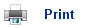# Partition Equilibria

• In addition to phase equilibria (between gaseous and liquid state), and chemical equilibria, there is also an equilibrium that exists between solutes in different solvents.
• Iodine is a Group VII liquid that dissolves in both inorganic and organic solvents, such as potassium iodide and tetrachloromethane.
• When iodine is dissolved in potassium iodide, the solution is brown in colour: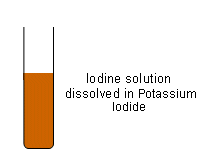• If this solution is then added to a separating funnel with CCl4, the iodine passes between the two liquids until an equilibrium is established: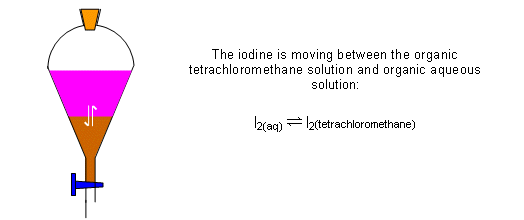• The layers could be run into separate containers and the concentration of iodine in each solvent determined: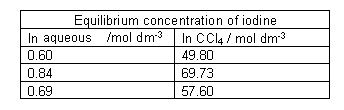• This can be written as an equilibrium constant: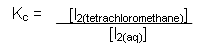• The value for the equilibrium constant comes out at around 84. This particular kind of equilibrium constant measures how a substance distributes (partitions) itself between two solvents. It is called a partition coefficient Kow.
• The partition coefficient in the above example is determined by the fact that iodine is a non-polar substance and therefore prefers to be dissolved in an organic substance.

## Useful books for revision

Revise A2 Chemistry for Salters (OCR A Level Chemistry B)Salters (OCR) Revise A2 ChemistryHome